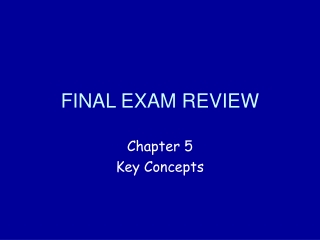# FINAL EXAM REVIEW - PowerPoint PPT PresentationDownload PresentationFINAL EXAM REVIEW

FINAL EXAM REVIEW
Download Presentation## FINAL EXAM REVIEW

- - - - - - - - - - - - - - - - - - - - - - - - - - - E N D - - - - - - - - - - - - - - - - - - - - - - - - - - -
##### Presentation Transcript

1. FINAL EXAM REVIEW Chapter 5 Key Concepts

2. Chapter 5 Vocabulary parallelogram • opposite sides • opposite angles • diagonals rectangle rhombus square trapezoid

3. Defn. of a Parallelogram A quadrilateral with both pairs of opposite sides parallel A B ABCD, In AB DC and AD BC D C

4. Theorem Opposite sides of a parallelogram are congruent.

5. Theorem Opposite angles of a parallelogram are congruent.

6. Theorem Diagonals of a parallelogram bisect each other.

7. Theorem ~ One pair of opp. sides both // and = Parallelogram

8. The Five Ways to Show a Quadrilateral is a Parallelogram 1) Defn. of ~ 2) Both pairs of opp. sides = ~ 3) One pair of opp. sides both // and = ~ 7 4) Both pairs of opp. ‘s = 5) Diagonals bisect each other

9. Theorem If 2 lines are parallel, then all points on one line are equidistant from the other line. A B AC = BD l m C D

10. Theorem If 3 parallel lines cut off congruent segments on one transversal, then they cut off congruent segments on every transversal. If AB = BC then XY = YZ A X B Y C Z

11. Theorem A line that contains the midpoint of one side of a triangle and is parallel to another side passes through the midpoint of the third side. X If M is the midpoint of XY then N is the midpoint of XZ N M Y Z

12. Theorem A segment that joins the midpoints of 2 sides of a triangle; 1. is parallel to the third side. 2. is half as long as the third side. X • MN YZ • MN = ½YZ N M Y Z

13. Theorem: The diagonals of a rectangle are congruent

14. Theorem: The diagonals of a rhombus are perpendicular.

15. Theorem: The midpoint of the hypotenuse of a right triangle is equidistant from all three vertices. .

16. Theorem: If an angle of a parallelogram is a right angle, then the parallelogram is a rectangle.

17. Theorem: If two consecutive sides of a parallelogram are congruent, then the parallelogram is a rhombus.

18. Trapezoid (Defn.) A quadrilateral with exactly one pair of parallel sides. leg base base leg

19. IsoscelesTrapezoid (Defn.) A trapezoid with congruent legs.

20. Theorem Base angles of an isosceles trapezoid are congruent.

21. Theorem The median of a trapezoid: 1. is parallel to the bases. 2. has a length equal to the average of the base lengths. A B XY = ½(AB + CD) median X Y C D

22. Homework • Chapter 5 Review WS